# 【机器学习】从SVM到SVR

：最近在工作中，高频率的接触到了SVM模型，而且还有使用SVM模型做回归的情况，即SVR。另外考虑到自己从第一次知道这个模型到现在也差不多两年时间了，从最开始的腾云驾雾到现在有了一点直观的认识，花费了不少时间。因此在这里做个总结，比较一下使用同一个模型做分类和回归之间的差别，也纪念一下与SVM相遇的两周年！这篇总结，不会涉及太多公式，只是希望通过可视化的方法对SVM有一个比较直观的认识。

### 0. 支持向量机（support vector machine, SVM）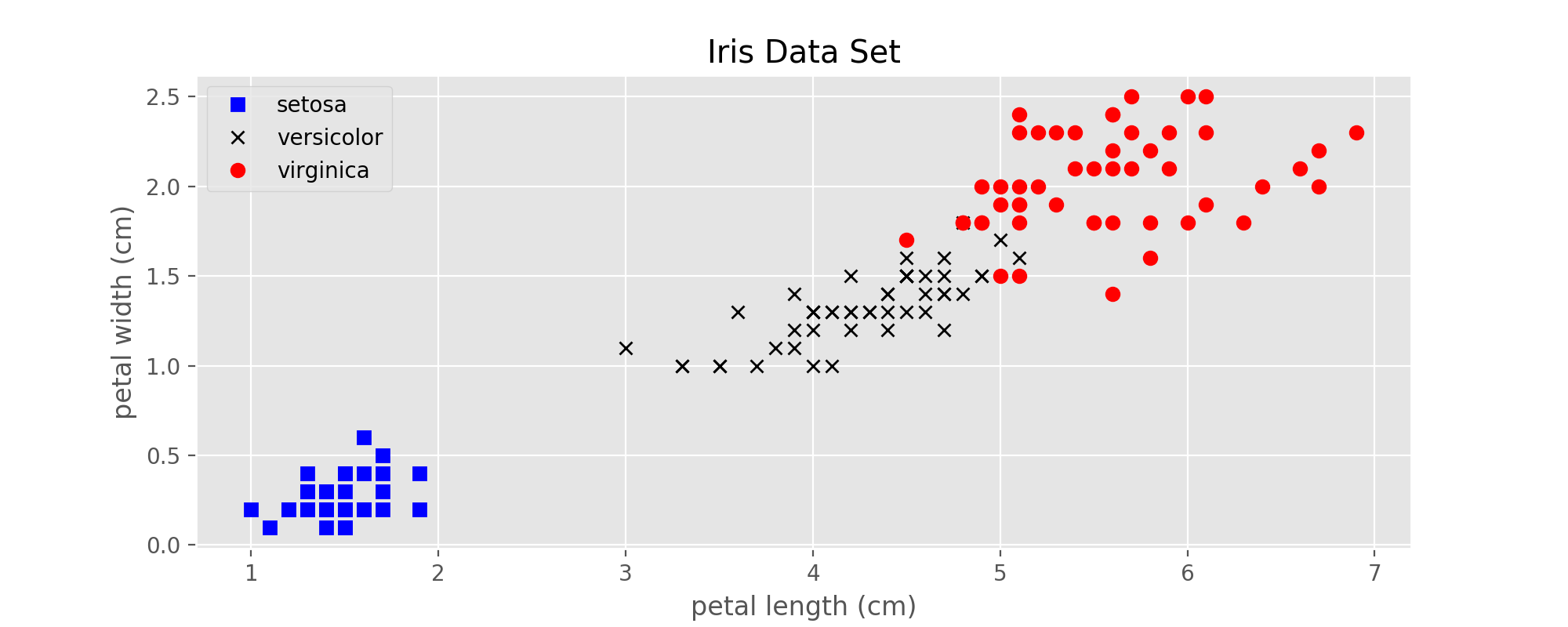### 1. SVM的前身：感知机（Perceptron）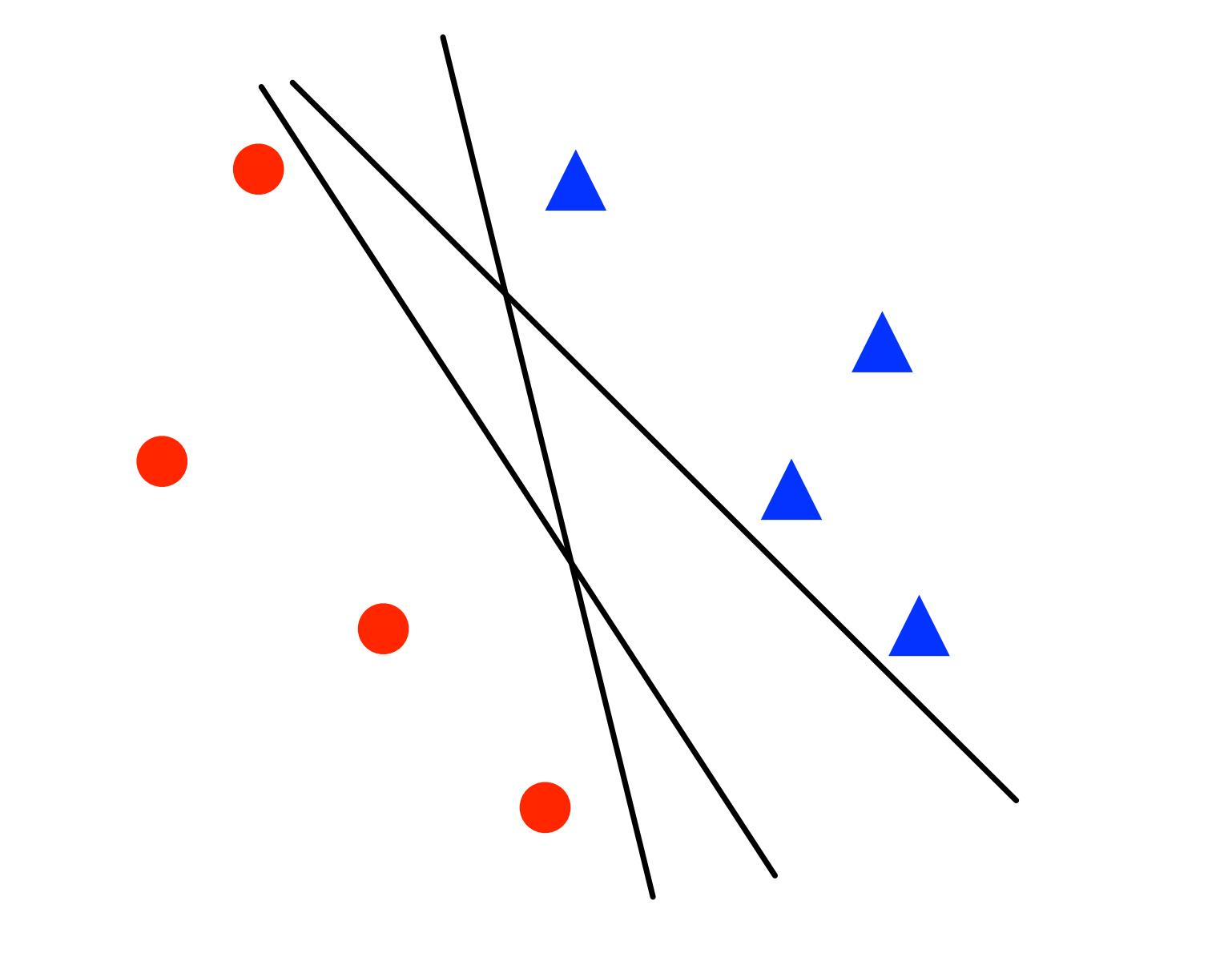图2：感知机训练出来的3个不同的线性分类器

#### 1.1 对二分类问题的具体描述

• 参数: $w \in \mathbb{ R }^n$ and $b \in \mathbb{ R }$
• 决策边界（Decision boundary）：$w \cdot x + b = 0$
• 对于一个新的点$x$做分类时，预测标签为$sign(w \cdot x + b)$

#### 1.3 算法的流程

step1: 初始化$w = 0, b = 0$;

step2: 循环从训练集取样本，每次一个

if $y (w \cdot x + b) \leq 0$（该样本分类错误）:

w = w + yx

b = b + y

### 2. SVM - 线性可分

#### 2.1 SVM的代价函数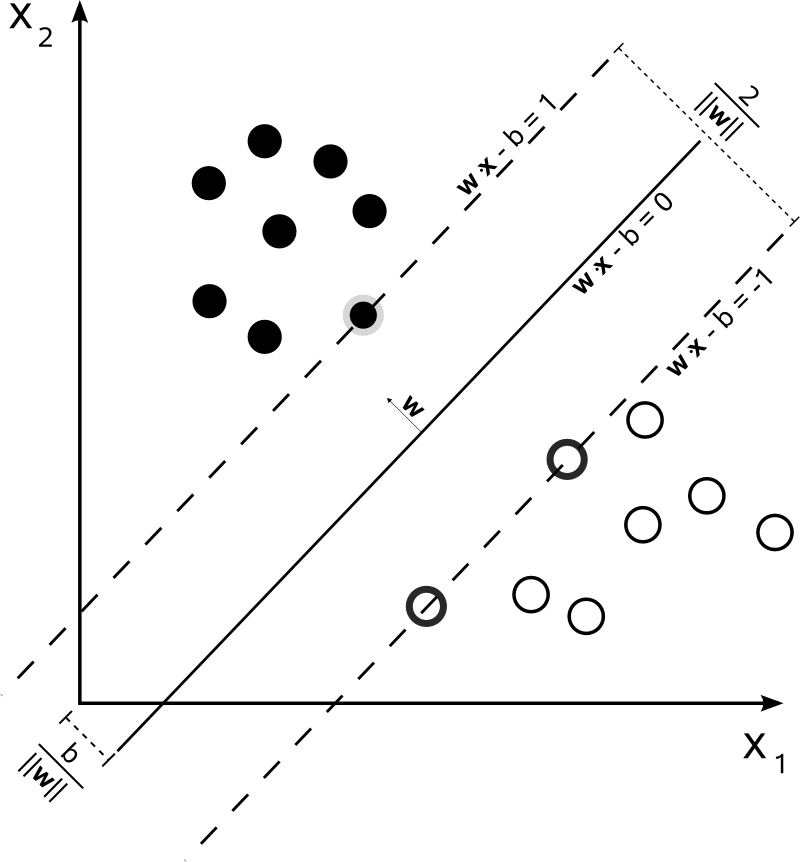#### 2.2 决策边界以及间隔

• 两条虚线之间的距离为$\frac{2}{||w||}$；
• 待优化参数$w$的方向就是决策边界的法向量方向（$w$与决策边界垂直）；
• 此时边界上一共有3个点，这三个点也就是此时的支持向量。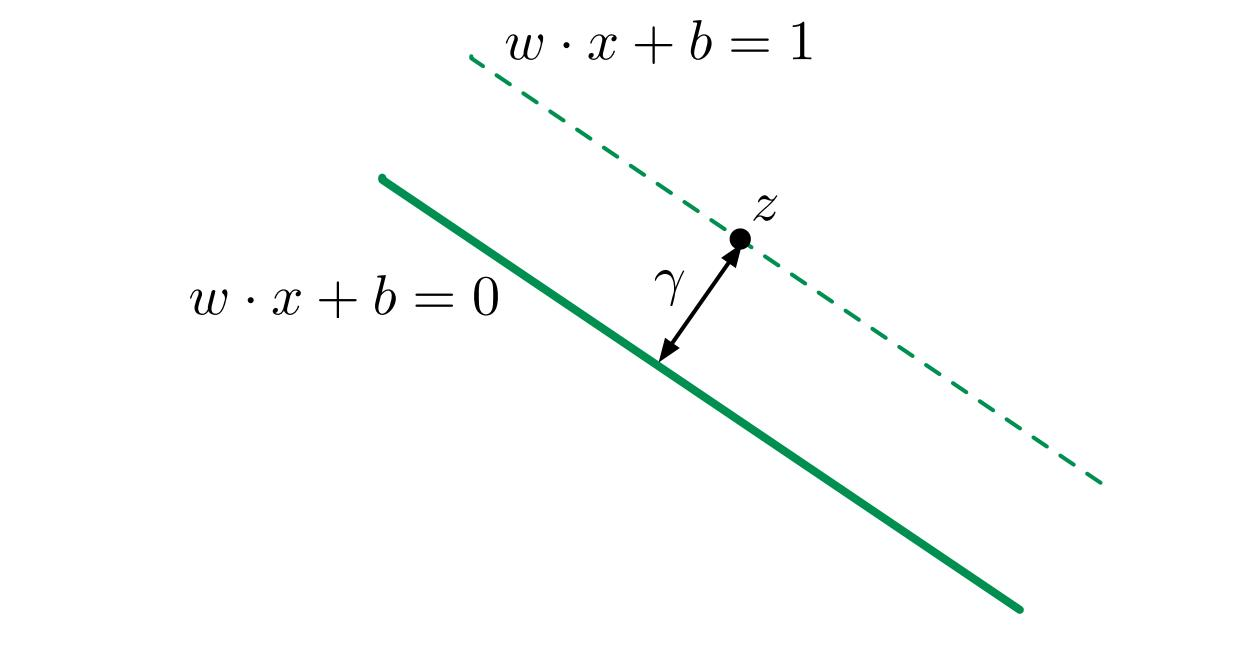#### 2.3 优化目标

s.t. $y^{(i)}(w \cdot x^{(i)} + b) ≥ 1$对于所有的$i = 1, 2, ..., m$成立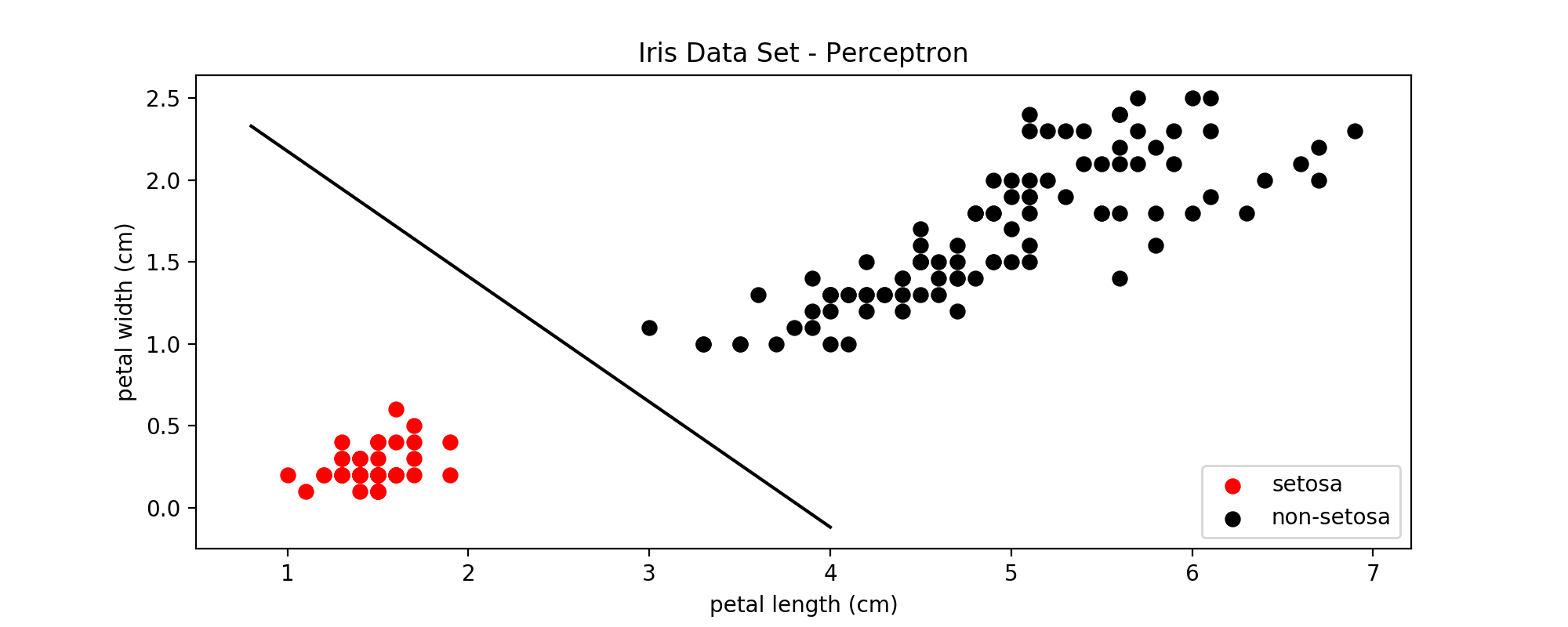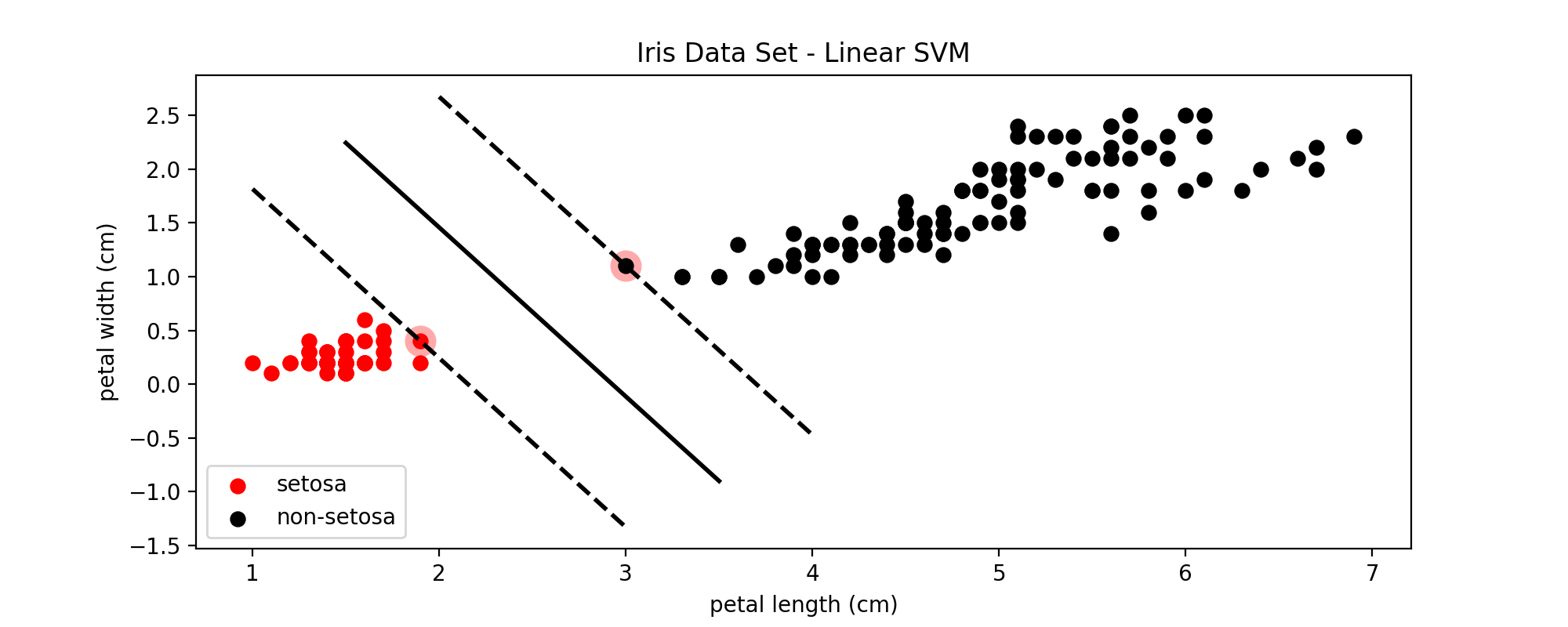### 3. SVM - 线性不可分

s.t. $y^{(i)}(w \cdot x^{(i)} + b) ≥ 1 - \xi_i$对于所有的$i = 1, 2, ..., m$成立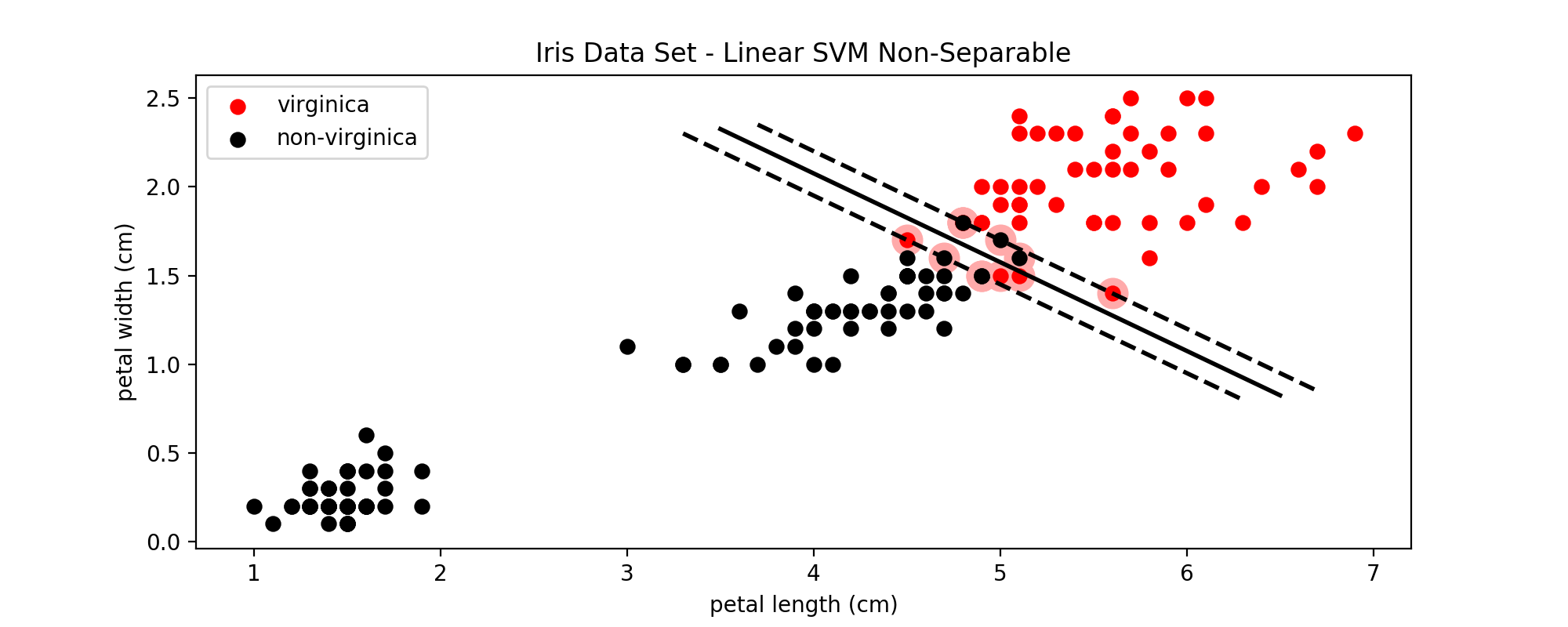C作为SVM模型的超参数之一，需要从一个较大的范围中一步一步的筛选，直到找到最适合的C。C值越大，表示错误分类的代价越大，就越趋于拒绝错误分类，即hard margin；C值越小，表示错误分类的代价越小，就越能容忍错误分类，即soft margin。即使是在线性可分的情况下，如果C设置的非常小，也可能导致错误分类的出现；在线性不可分的情况下，设置过大的C值会导致训练无法收敛。

### 4. SVR - 利用SVM做回归分析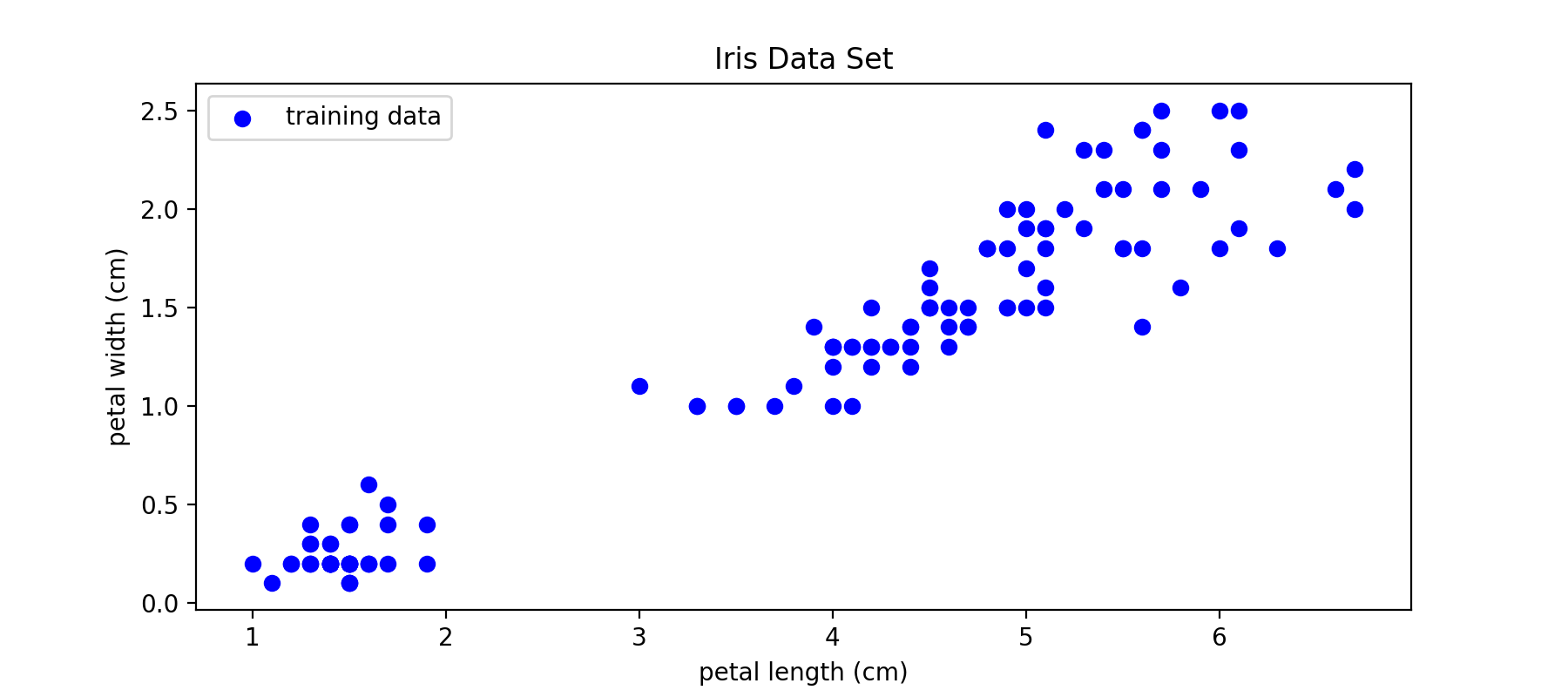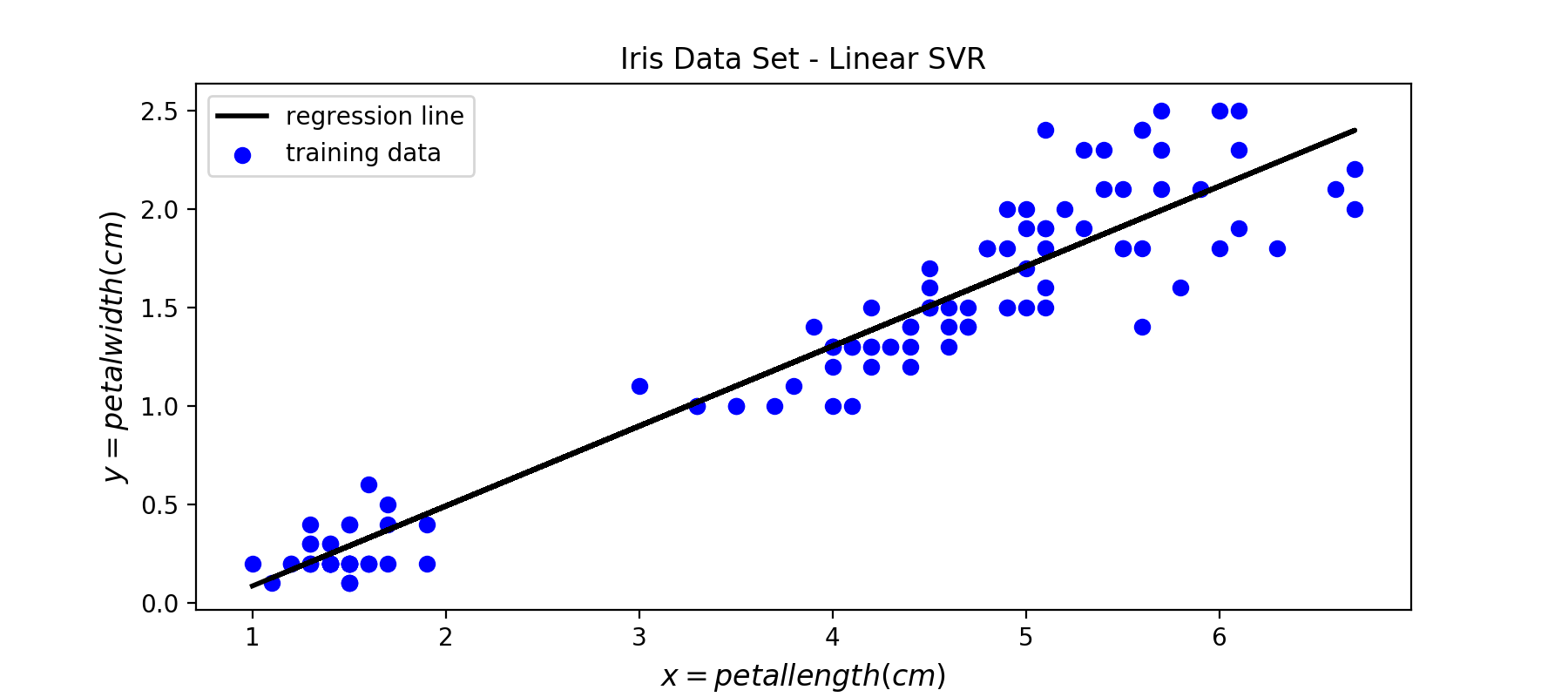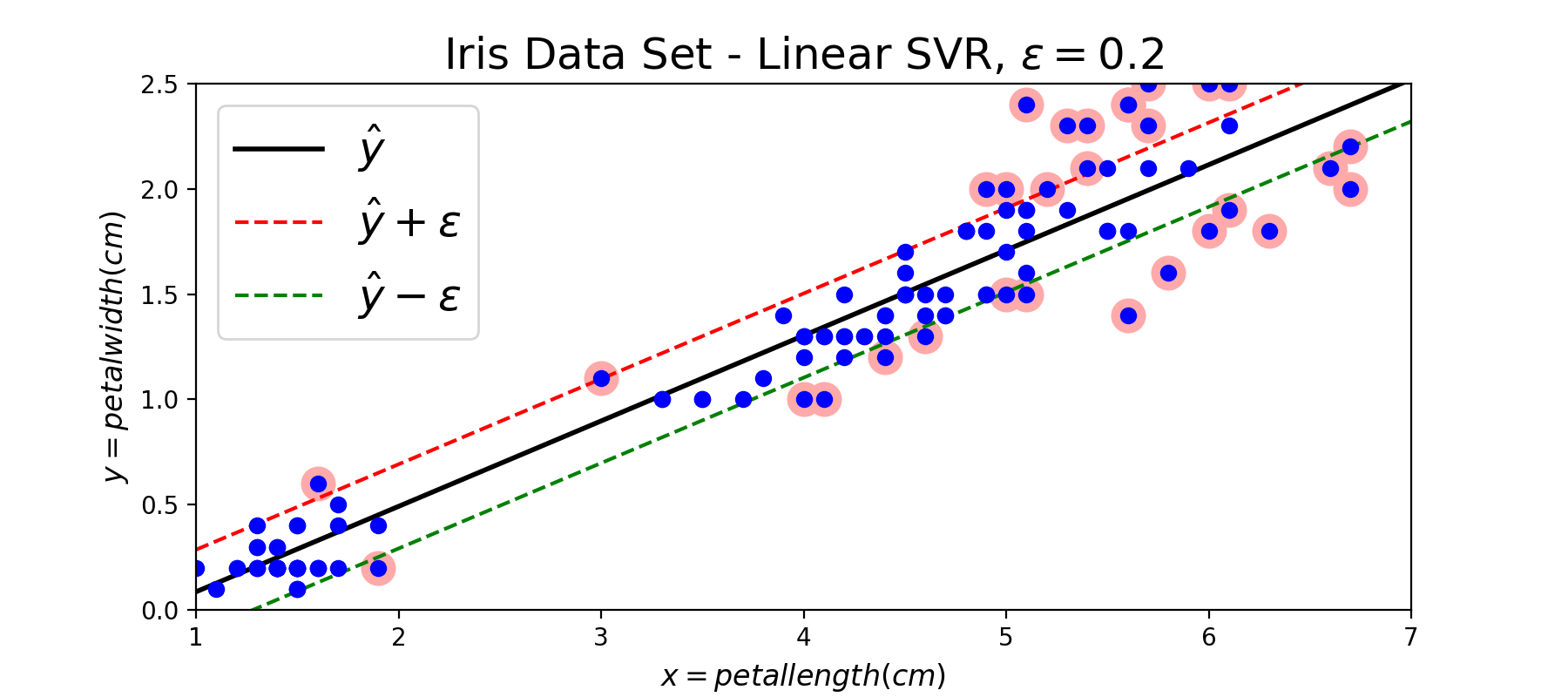### Reference

https://zh.wikipedia.org/wiki/%E6%94%AF%E6%8C%81%E5%90%91%E9%87%8F%E6%9C%BA

https://zhuanlan.zhihu.com/p/26263309, 直线方程的各种形式

https://github.com/ageron/handson-ml/blob/master/05_support_vector_machines.ipynb

http://www.svms.org/regression/SmSc98.pdf

http://www.robots.ox.ac.uk/~az/lectures/ml/

edx: UCSanDiegoX - DSE220x Machine Learning Fundamentals

https://github.com/OnlyBelter/jupyter-note/blob/master/machine_learning/SVM/04_how%20SVM%20becomes%20to%20SVR.ipynb, 文中代码

2020.12.19 typo fix

posted @ 2018-05-01 20:12  昕-2008  阅读(36035)  评论(0编辑  收藏  举报